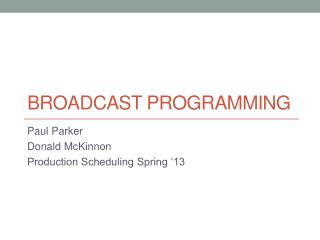# Broadcast Programming - PowerPoint PPT PresentationDownload PresentationDownload Presentation- - - - - - - - - - - - - - - - - - - - - - - - - - - E N D - - - - - - - - - - - - - - - - - - - - - - - - - - -
##### Presentation Transcript

1. Broadcast Programming Paul Parker Donald McKinnon Production Scheduling Spring ‘13

2. Agenda • Problem Overview • Our Solution • Analysis • Conclusion • Extensions

3. Problem Overview (1) Description: Schedule shows of equal duration such that the sum of the viewers over all periods is greatest. Given: The number of viewers a shows garners is dependent onits time period and neighbors. Example: Schedule 48 different 30 minute shows in a 24 hour period. Maximize the sum of viewers over all periods, where number of viewers of the show in period 35 depends on the 35th period and the shows in periods 34 and 36.

4. Problem Overview (2) Upper Bound: The sum of the maximum number of viewers any show can have over each time slot (typically infeasible to schedule). NP Hard: Broadcast Programming reduces Knapsack Assumptions • All jobs (shows) have the same duration. • Unscheduled jobs gain no viewers. • Jobs can only occur in 1 period in a schedule (no reruns).

5. Our Solution: Ratio Based Swapping Objective: Given n shows, m periods, and function(ni-1,ni,ni+1,mj)= vi,j, viewers for show niin period mj, max Σj(vi,j), where i=1…n, and j=1…m. Algorithm: • Randomly schedule shows until all mtime slots have a distinct show, or all shows are scheduled. • Determine the show S with the smallest ratio of its viewers in its current period, P, to its average viewers in all periods of the current schedule: vS,P÷ AVG(all vS,j)where j=1…m. • If S can be swapped with another period such that the sum of the viewers over all periods increases, then swap Ssuch that the greatest increase in viewers results and go to Step 2. Otherwise, STOP and output the objective value of the current schedule.

6. Analysis – Function 1 Vi,j = 100 * [1 + sine(Si,j-1* Si,j* Si,j+1)], where i=1…n, and j=1…m *Simulations done in MATLAB or Java

7. Analysis – Function 2 Vi,j = Si,j-1 * Si,j * Si,j+1, where i=1…n, and j=1…m *Simulations done in MATLAB or Java

8. Analysis – Function 3 Vi,j = j * (Si,j-1 * Si,j * Si,j+1), where i=1…n, and j=1…m *Simulations done in MATLAB or Java

9. Conclusion Our Heuristic: • Consistency Average objective value is good (above average) • Efficiency Good objective values result after few swaps • Scalability Yields high objective values given different viewership functions

10. Algorithmic Extensions Considered • Derive initial schedule as follows • Schedule the show and its neighbors that will garner the most viewers in the remaining time slot until there are no empty time slots remaining. • Expanded search after finding local maximum • When a maximum objective value is achieved, swap the two jobs in the time slots having the least viewers and resolve this instance, disallowing the algorithm to re-swap these two jobs immediately. • Advertisements • Model advertisements for shows as shorter jobs and schedule them (not necessarily adjacent to the show they promote) to maximize viewership.

11. Heuristic for Initial Schedule Configuration • Results • Inconclusive • Possible Implication: *Simulations done in MATLAB or Java

12. Thank You